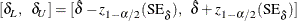# The LIFEREG Procedure

### Confidence Intervals

Subsections:

Confidence intervals are computed for all model parameters and are reported in the "Analysis of Parameter Estimates" table. The confidence coefficient can be specified with the ALPHA=MODEL statement option, resulting in a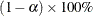two-sided confidence coefficient. The default confidence coefficient is 95%, corresponding to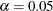.

#### Regression Parameters

A two-sidedconfidence interval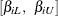for the regression parameter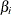is based on the asymptotic normality of the maximum likelihood estimator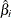and is computed by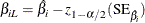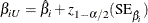where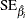is the estimated standard error of, and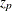is the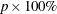percentile of the standard normal distribution.

#### Scale Parameter

A two-sidedconfidence interval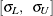for the scale parameterin the location-scale model is based on the asymptotic normality of the logarithm of the maximum likelihood estimator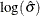, and is computed by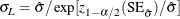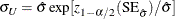See Meeker and Escobar (1998) for more information.

#### Weibull Scale and Shape Parameters

The Weibull distribution scale parameter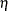and shape parameter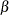are obtained by transforming the extreme-value location parameterand scale parameter: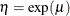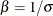Consequently, two-sidedconfidence intervals for the Weibull scale and shape parameters are computed as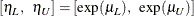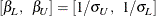#### Gamma Shape Parameter

A two-sidedconfidence interval for the three-parameter gamma shape parameter
is computed by